# Algorithms for Algebraic Number Theory

I think the best overall reference for algorithms for doing basic algebraic number theory computations is [Coh93].

Our main long-term algorithmic goals for this book (which we won't succeed at reaching) are to understand good algorithms for solving the following problems in particular cases:

• Ring of integers: Given a number field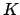(by giving a polynomial), compute the full ring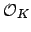of integers.
• Decomposition of primes: Given a prime number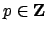, find the decomposition of the ideal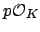as a product of prime ideals of.
• Class group: Compute the group of equivalence classes of nonzero ideals of, where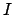and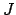are equivalent if there existssuch that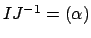.
• Units: Compute generators for the group of units of.

As we will see, somewhat surprisingly it turns out that algorithmically by far the most time-consuming step in computing the ring of integersseems to be to factor the discriminant of a polynomial whose root generates the field. The algorithm(s) for computingare quite complicated to describe, but the first step is to factor this discriminant, and it takes much longer in practice than all the other complicated steps.

William Stein 2004-05-06